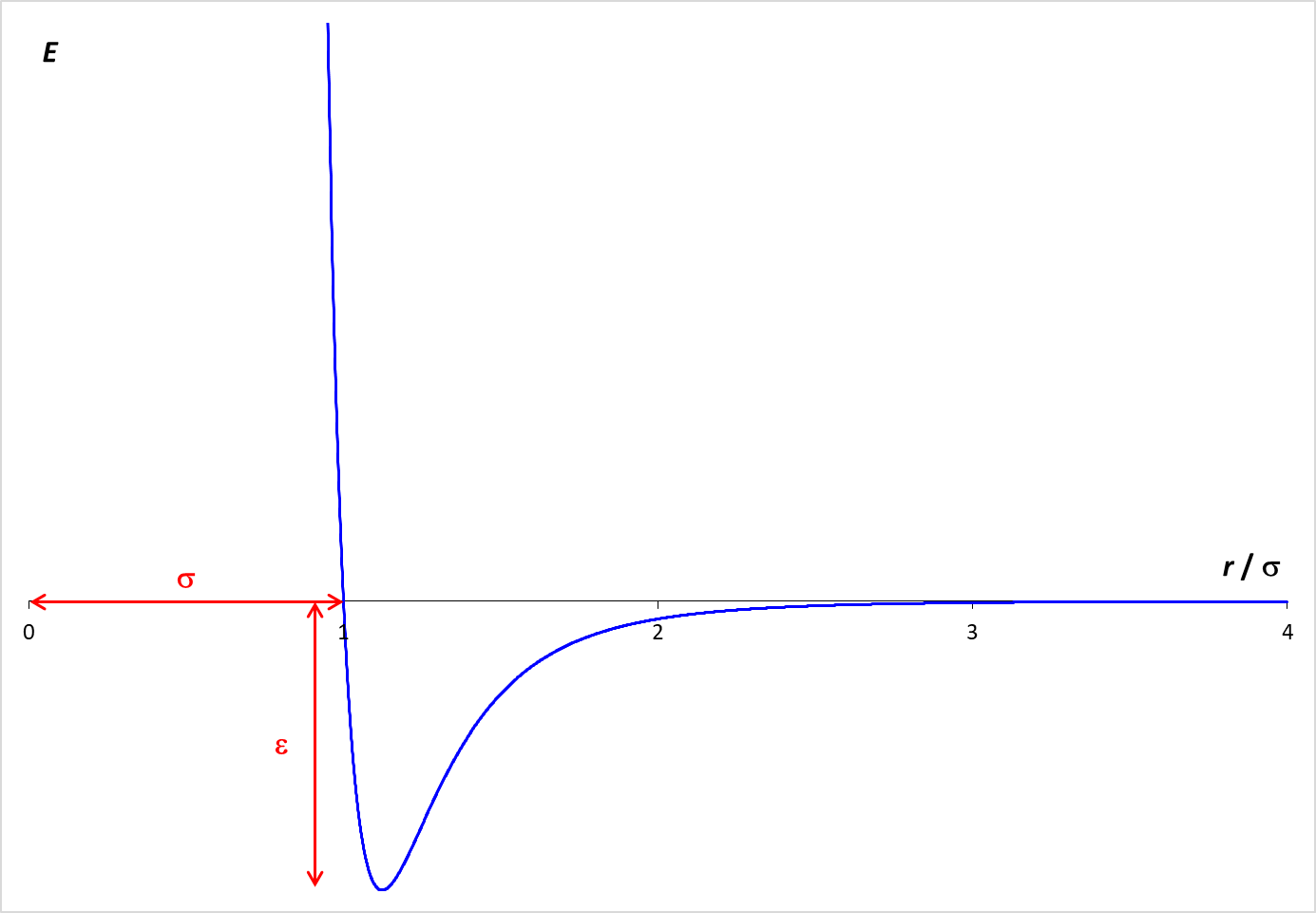# Molecular Dynamics Simulation: The Liquid Phase

Statistical mechanics envisions matter as being composed of a large number of small particles in rapid motions. A simulation of this behavior is provided below. This simulation, called Molecular Dynamics, applies Newton's laws of motion to predict the paths of individual particles or molecules, represented by the red balls. The forces that operate on the particles are described by the Lennard-Jones potential, which is graphed at the right. The graph shows how the potential energy (PE) arising from the interaction of two particles varies with the distance (r) between the particles.

When the molecules are far apart, the PE is essentially zero.
As the molecules get closer, there is an attractive force between the particles, which lowers the PE. The particles need to be fairly close for this attraction to be relevant. In the simulation, the particle diameter is σ. Examination of the graph shows that the particles need to be within about two diameters (2 σ ) for a significant attractive force to exist. If the molecules get too close (within one diameter), however, the PE increases rapidly. This strong repulsive force drives particles away from each other and is the reason why the particle "bounce" off each other.

The depth of the Lennard-Jones energy well is ε which is 1.0 kJ/mol in this simulation.## Questions

Run this simulation of a liquid, observe the behavior of the molecules, and answer the following questions.
1. What features are characteristic of the liquid phase?
1. Does the liquid have a rigid shape? Does the shape ever change?
2. Does every molecule freely move to all regions of the container?
3. Is the system completely filled by the liquid or does the liquid only occupy part of the container?
4. Which molecules are in the gas phase and which are in the liquid phase?

2. Describe how the molecules move.
1. Are the molecules in the liquid stationary or do they move?
2. Do molecules within the liquid move freely throughout the liquid?
3. Are the molecules packed randomly in the liquid or is there a systematic pattern to the packing of the molecules?

3. Describe the interactions between molecules.
1. Why are the molecules unable to move away from each other?
2. Is it possible for a single molecule to occasionally escape from the liquid?
3. Is the average potential energy positive or negative? Explain the significance of the sign of the potential energy.
4. Is the magnitude of the average potential energy relatively small or large? Explain why.

Properties

K
200 - 1000 K

mol/L

bar

Two-Dimensions

kJ/mol

kJ/mol

Simulation Control

Molecular Dynamics - The Liquid Phase (MDS-Liquid.html, version 1.0)